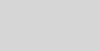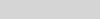# Functions View for Build Insights in Visual Studio 2022 17.8Eve Silfanus

### Introduction

We are excited to unveil a new feature in Build Insights for Visual Studio: Functions View! This feature is available in Visual Studio 2022 version 17.8. Functions View offers essential insights into functions and forceinlines within your codebases.

We extend our sincere thanks thanks to the developer community, especially our game studio partners, for actively providing feedback. Your contributions are invaluable in shaping this new feature.

For more details about Build Insights and to explore other features like Included Files and Include Tree Views, please visit our initial announcement blogpost.

### Code Generation Insights with Functions View

Functions View is a powerful tool that displays the impact of each function on the total build time by analyzing code generation times and forceinlines. Forceinlines, commonly used to boost runtime efficiency, can also influence build times.

The following sample code is based on a public code sample by Aras Pranckevičius. We will use it to show you how you can optimize your builds with Functions View. To setup your project, create a C++ Console application and copy the following sample code:

``````#include <emmintrin.h>

struct float4
{
__m128 val;
float4() { val = _mm_setzero_ps(); }
float4(float x) { val = _mm_set1_ps(x); }
float4(float x, float y) { val = _mm_set_ps(y, x, y, x); }
float4(float x, float y, float z) { val = _mm_set_ps(0.f, z, y, x); }
float4(float x, float y, float z, float w) { val = _mm_set_ps(w, z, y, x); }
float4(__m128 v) { val = v; }
};

static __forceinline float4 operator+(const float4& a, const float4& b) { return float4(_mm_add_ps(a.val, b.val)); }
static __forceinline float4 operator-(const float4& a, const float4& b) { return float4(_mm_sub_ps(a.val, b.val)); }
static __forceinline float4 operator*(const float4& a, const float4& b) { return float4(_mm_mul_ps(a.val, b.val)); }
static __forceinline float4 operator/(const float4& a, const float4& b) { return float4(_mm_div_ps(a.val, b.val)); }
static __forceinline float4 csum(const float4& p)
{
__m128 r = _mm_add_ps(p.val, _mm_castsi128_ps(_mm_shuffle_epi32(_mm_castps_si128(p.val), _MM_SHUFFLE(0, 3, 2, 1))));
return _mm_add_ps(r, _mm_castsi128_ps(_mm_shuffle_epi32(_mm_castps_si128(r), _MM_SHUFFLE(1, 0, 3, 2))));
}
static __forceinline float4 dot(const float4& p0, const float4& p1) { return csum(p0 * p1); }
static __forceinline float4 dot(const float4& p) { return dot(p, p); }
static __forceinline float4 rsqrt(const float4& x)
{
#define C0  9.999998e-01f
#define C1  3.0000002e+00f
#define C2  .5f
#define C3  340282346638528859811704183484516925440.f
__m128 e = _mm_mul_ps(_mm_rsqrt_ps((__m128) x.val), _mm_set_ps(C0, C0, C0, C0));
e = _mm_min_ps(e, _mm_set_ps(C3, C3, C3, C3));
return _mm_mul_ps(_mm_mul_ps(e, _mm_set_ps(C2, C2, C2, C2)), _mm_sub_ps(_mm_set_ps(C1, C1, C1, C1), _mm_mul_ps(_mm_mul_ps(x.val, e), e)));
}
static __forceinline float4 normalize(const float4& v)
{
return v * rsqrt(dot(v));
}

static __forceinline float4 ident() { return float4(0.f, 0.f, 0.f, 1.f); }
static __forceinline float4 sampleFun1(const float4& x, const float4& y)
{
return csum(x) / x + y;
}
static __forceinline float4 sampleFun2(const float4& q1, const float4& q2)
{
return sampleFun1(q1 * q2, q2 - q1) * (q1 + q2);
}
static float4 sampleFun3(const float4& pq, const float4& mask)
{
const float c8 = 0.923879532511287f;
const float s8 = 0.38268343236509f;
const float g = 5.82842712474619f;

float4 ch = float4(2) * (normalize(pq) - normalize(mask));
float4 sh = pq * normalize(ch);
float4 r = ((g * sh * sh - ch * ch) + sh / float4(s8, s8, s8, c8)) * mask;
return normalize(r);
}
struct matrix
{
float4 m0, m1, m2;
};
static __forceinline float4 sampleFunIteration(matrix& s, int count = 5)
{
matrix qm;
float4 q, v = ident();
for (int iter = 0; iter < count; iter++)
{
q = sampleFun3(s.m0, float4(0, 0, 1, 1));
v = sampleFun2(v, q);
v = normalize(v);
q = sampleFun3(s.m1, float4(1, 0, 0, 1));
v = sampleFun2(v, q);
v = normalize(v);
q = sampleFun3(s.m2, float4(0, 1, 0, 1));
v = sampleFun2(v, q);
v = normalize(v);
}
return v;
}

static __forceinline float4 sampleFunDecomposition(const matrix& a, const float4& u, const float4& v)
{
float4 r;
matrix s = a;
s.m0 = normalize(s.m0) + u;
s.m1 = normalize(s.m1) * v;
s.m2 = normalize(s.m2);
r = sampleFunIteration(s);
r = normalize(v) * u + (normalize(u) / v);
s.m0 = s.m0 + r;
s.m1 = s.m1 + r;
s.m2 = s.m2 + r;
r = sampleFunIteration(s);
s.m0 = s.m0 / normalize(r);
s.m1 = s.m1 / normalize(v + r);
s.m2 = s.m2 / normalize(v * r);
r = sampleFunIteration(s);
s.m0 = s.m0 * s.m1;
s.m1 = s.m1 * s.m2 - r;
s.m2 = s.m2 * s.m0 + r;
r = sampleFunIteration(s);

return r;
}

int main(int argc, const char** argv)
{
matrix a;
a.m0 = (float)argv;
a.m1 = (float)argc;
a.m2 = (float)(argv - argc);

float4 u = a.m0;
float4 v = a.m1;
float4 e = sampleFunDecomposition(a, a.m0, a.m1);
e = e + sampleFunDecomposition(a, a.m2, normalize(a.m1));
e = e + sampleFunDecomposition(a, normalize(a.m2), e);
e = e + sampleFunDecomposition(a, e, e);
e = e + sampleFunDecomposition(a, normalize(e), normalize(e));
e = e * sampleFunDecomposition(a, e, e);
e = e - sampleFunDecomposition(a, e, e);
e = e * sampleFunDecomposition(a, e, e);
e = e + sampleFunDecomposition(a, e, e);
float4 r = normalize(e);

return (int)_mm_cvtss_f32(r.val);
}``````

Before running the sample code, ensure the functions will be forceinlined:

1. Right-click on the project in the solution explorer to open the context menu.
2. Select project properties.
3. In the Project Property Pages, navigate to C/C++ > Optimization.
4. Under Optimization, select “Maximum Optimization (Favor Speed) (/Ox)”
5. Click OK, then choose Release as the configuration.

Finally, under the Build Menu, select Run Build Insights on Solution > Build. The following is the report generated by Build Insights:The total build time is 7.342 seconds. The main function includes 8 instances of the forceinlined`sampleFunDecomposition()` function, significantly contributing to the main function’s size.

To mitigate the build time impact, remove the `__forceinline` from the `sampleFunDecomposition()` function and observe the effect on build time.

``````static float4 sampleFunDecomposition(const matrix& a, const float4& u, const float4& v)
{
float4 r;
matrix s = a;
s.m0 = normalize(s.m0) + u;
s.m1 = normalize(s.m1) * v;
s.m2 = normalize(s.m2);
r = sampleFunIteration(s);
r = normalize(v) * u + (normalize(u) / v);
s.m0 = s.m0 + r;
s.m1 = s.m1 + r;
s.m2 = s.m2 + r;
r = sampleFunIteration(s);
s.m0 = s.m0 / normalize(r);
s.m1 = s.m1 / normalize(v + r);
s.m2 = s.m2 / normalize(v * r);
r = sampleFunIteration(s);
s.m0 = s.m0 * s.m1;
s.m1 = s.m1 * s.m2 - r;
s.m2 = s.m2 * s.m0 + r;
r = sampleFunIteration(s);

return r;
}``````

Then, run Build Insights again. The following are the results we got:By merely removing the `__forceinline` from `sampleFunDecomposition()`, the build time went from 7.342 seconds to 0.251 seconds, which is a 97% reduction from the original!

We are rapidly iterating to deliver more value to you. Anticipate the following in our next release:

• The Time Column will be renamed to Contribution for clarity.
• Addition of a Forceinline Count Column.
• Aggregation of forceinline metrics: forceinlines will be grouped when expanding a function.
• Introduction of Tool origin, indicating where the code generation occurred.
• Addition of Filepath column and navigation: It will show the file location of each forceinlined function, allowing for easy navigation, with the option to go to the source file.
• New Duration Column, reflecting time without considering parallel operations.